## 5.6 Plotting with ggplot2

`ggplot2` is a plotting package that makes it simple to create complex plots from data stored in a data frame. It provides a programmatic interface for specifying what variables to plot, how they are displayed, and general visual properties. Therefore, we only need minimal changes if the underlying data change or if we decide to change from a bar plot to a scatterplot. This helps in creating publication quality plots with minimal amounts of adjustments and tweaking.

``library(ggplot2)``

`ggplot2` functions work best with data in the ‘long’ format, i.e., a column for every dimension, and a row for every observation. Well-structured data will save you lots of time when making figures with `ggplot2`

`ggplot2` graphics are built step by step by adding new elements. Adding layers in this fashion allows for extensive flexibility and customization of plots.

Each chart built with `ggplot2` must include the following

• Data

• Aesthetic mapping (aes)

• Describes how variables are mapped onto graphical attributes
• Visual attribute of data including x-y axes, color, fill, shape, and alpha
• Geometric objects (geom)

• Determines how values are rendered graphically, as bars (geom_bar), scatterplot (geom_point), line (geom_line), etc.

Thus, the template for graphic in ggplot2 is:

``````<DATA> %>%
ggplot(aes(<MAPPINGS>)) +
<GEOM_FUNCTION>()``````

Remember from the last lesson that the pipe operator `%>%` places the result of the previous line(s) into the first argument of the function. `ggplot()` is a function that expects a data frame to be the first argument. This allows for us to change from specifying the `data =` argument within the `ggplot` function and instead pipe the data into the function.

• use the `ggplot()` function and bind the plot to a specific data frame.
``yearly_count %>% ggplot()``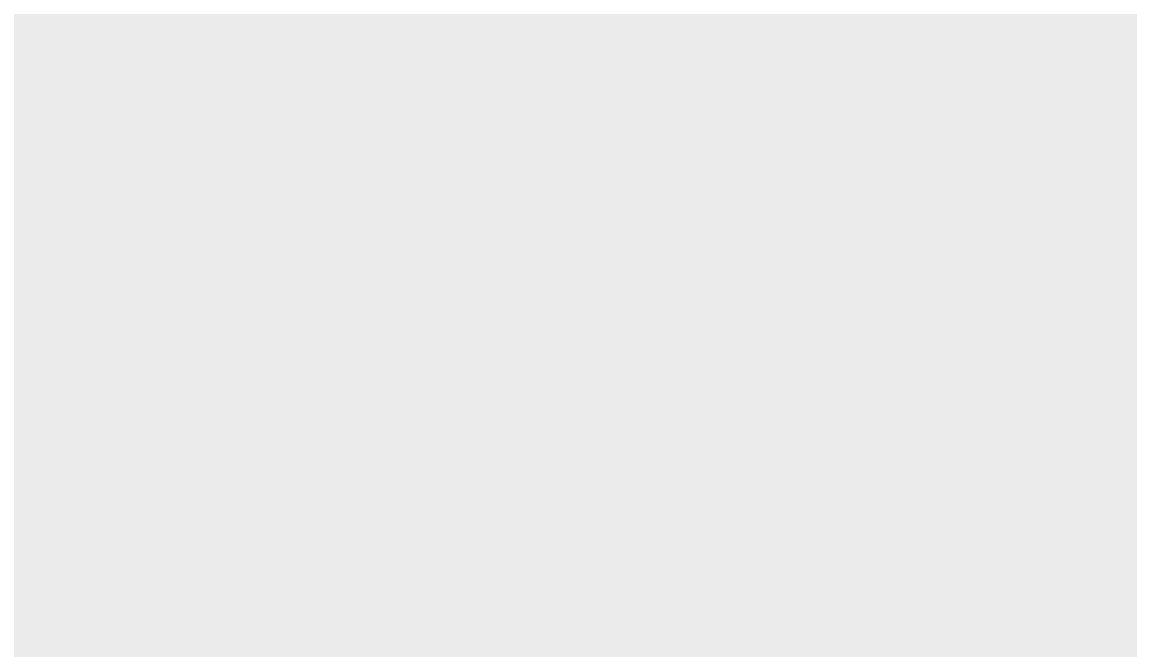Is the same as

``ggplot(data=yearly_count)``• define a mapping (using the aesthetic (`aes`) function), by selecting the variables to be plotted and specifying how to present them in the graph, e.g. as x/y positions or characteristics such as size, shape, color, etc.
``````yearly_count %>%
ggplot(aes(x=Year, y=TotalCount))``````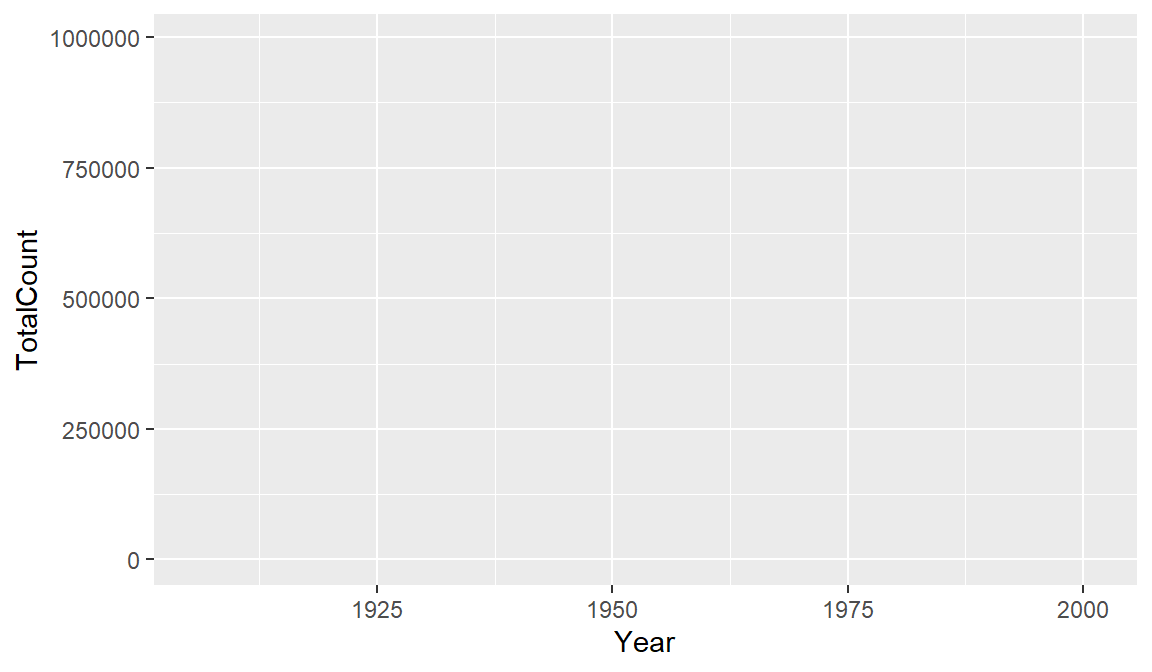This can also be written more concisely:

``````yearly_count %>%
ggplot(aes(Year, TotalCount))``````• add ‘geoms’ – graphical representations of the data in the plot (points, lines, bars). `ggplot2` offers many different geoms for common graph types. To add a geom to the plot use the `+` operator. Note to that you can save plots as objects.
``````year_total_line <-
yearly_count %>%
ggplot(aes(x=Year, y=TotalCount)) +
geom_line()

year_total_line``````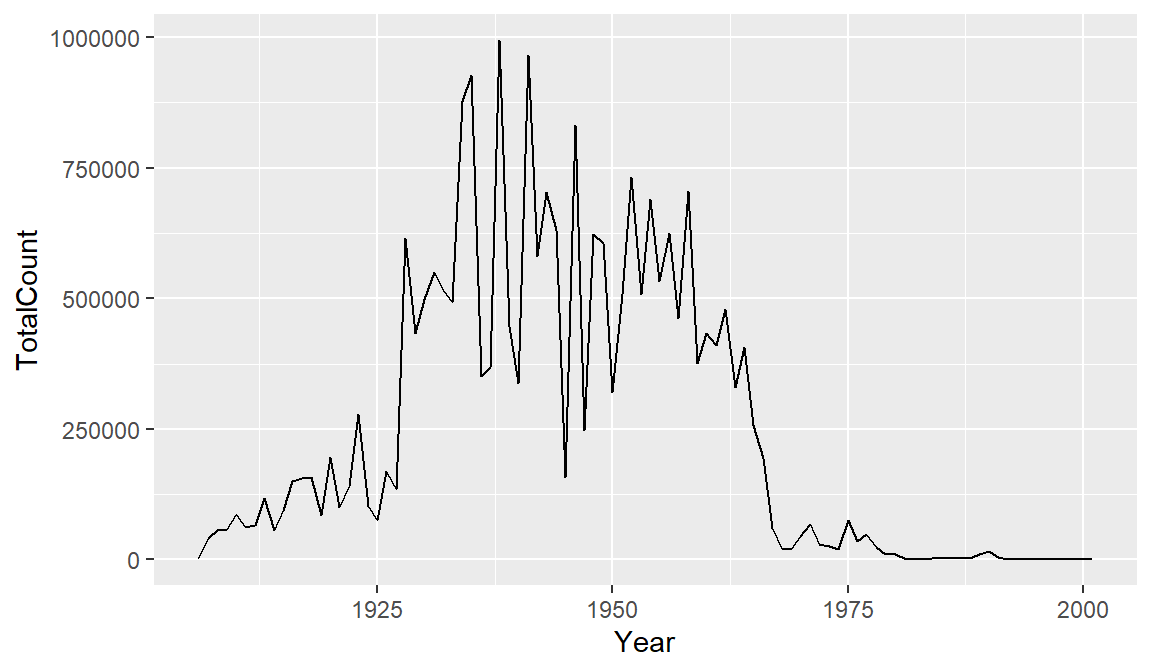It might be nice to see where each data point falls on the line. To do this we can add another geometry layer.

``````year_total_line <-
yearly_count %>%
ggplot(aes(x=Year, y=TotalCount)) +
geom_line() +
geom_point()

year_total_line``````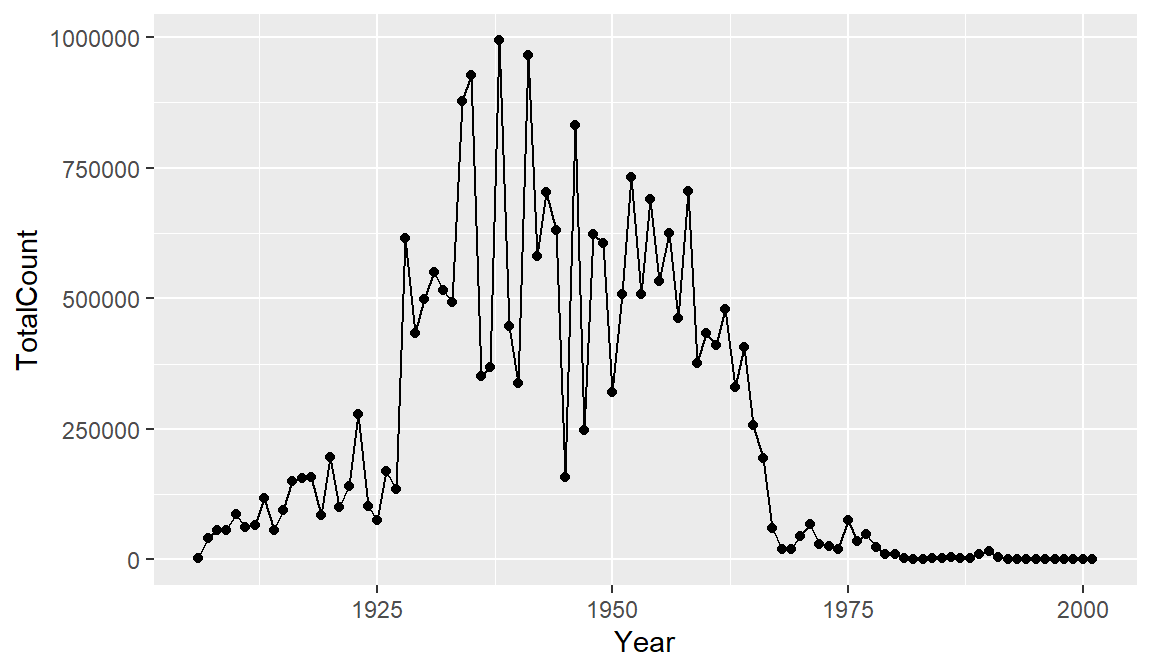The `+` in the `ggplot2` package is particularly useful because it allows you to modify existing `ggplot` objects. This means you can easily set up plot templates and conveniently explore different types of plots, so the above plot can also be generated with code like this:

``````year_total_line <- year_total_line + geom_point()

year_total_line``````There are many ways to customize your plot, like changing the color or line type, adding labels and annotations. One thing that would make our graph easier to read is tick marks at each decade on the x-axis.

``````year_total_line <-
yearly_count %>%
ggplot(aes(x=Year, y=TotalCount)) +
geom_line() +
geom_point() +
scale_x_continuous(breaks = seq(1900, 2000, 10))

year_total_line``````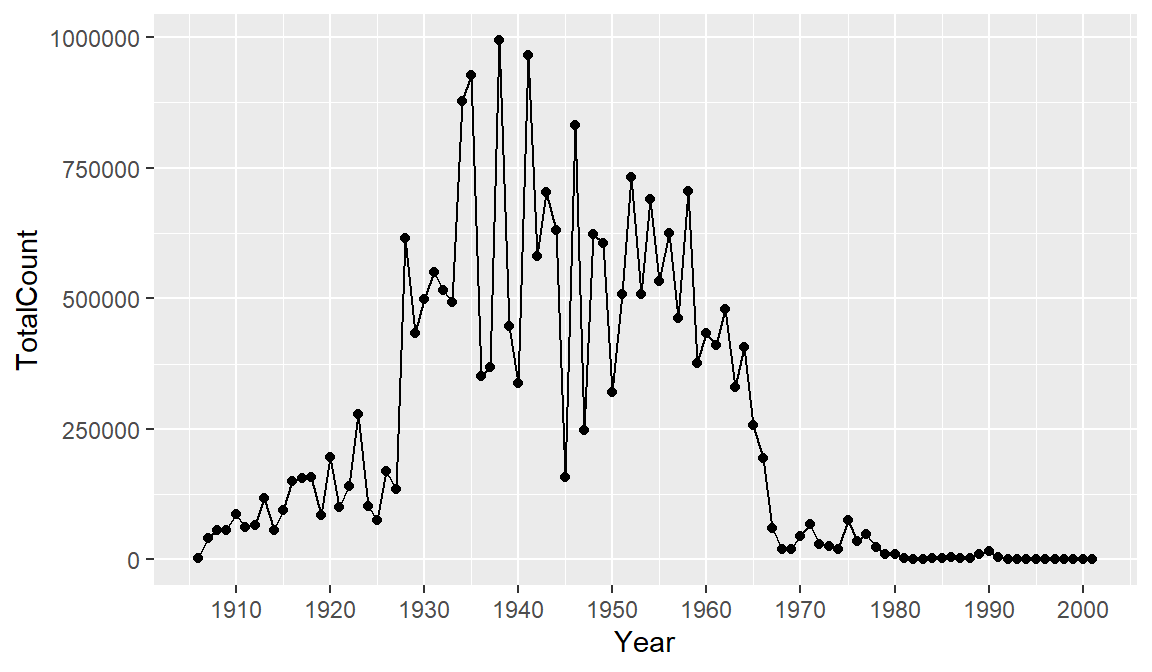Perhaps we would like to add some more information to our graph for context. Let’s drop a reference line at 1963 to clearly indicate on the graph when the vaccine was introduced. To do this we add a `geom_vline()` and the `annotate()` function. There are multiple ways of adding lines and text to a plot, but these will serve us well for this case. Note that you can change features of lines such as color, type, and size.

``````year_total_line <-
yearly_count %>%
ggplot(aes(x=Year, y=TotalCount)) +
geom_line() +
geom_point() +
scale_x_continuous(breaks = seq(1900, 2000, 10)) +
geom_vline(xintercept = 1963, color = "red", linetype= "dashed") +
annotate(geom = "label", x=1963, y=80000, label="1963: vaccine introduced")

year_total_line``````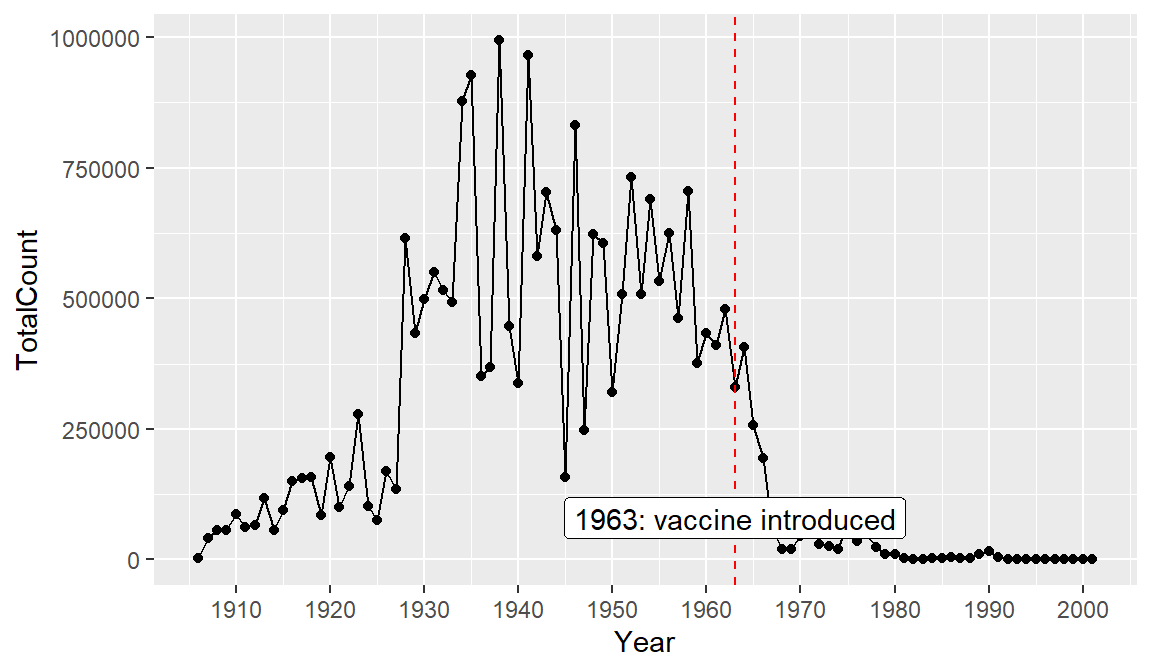Finally, let’s add a title and axis labels to our plot with the `labs()` function. Note that axis labels will automatically be supplied from the column names, but you can use this function to override those defaults.

``````year_total_line <-
yearly_count %>%
ggplot(aes(x=Year, y=TotalCount)) +
geom_line() +
geom_point() +
scale_x_continuous(breaks = seq(1900, 2000, 10)) +
geom_vline(xintercept = 1963, color = "red", linetype= "dashed") +
annotate(geom = "label", x=1963, y=800000, label="1963: vaccine introduced") +
labs(title = "Measles Cases Decrease After Vaccine Introduced", x = "Year", y = "Total Measles Case Count")

year_total_line``````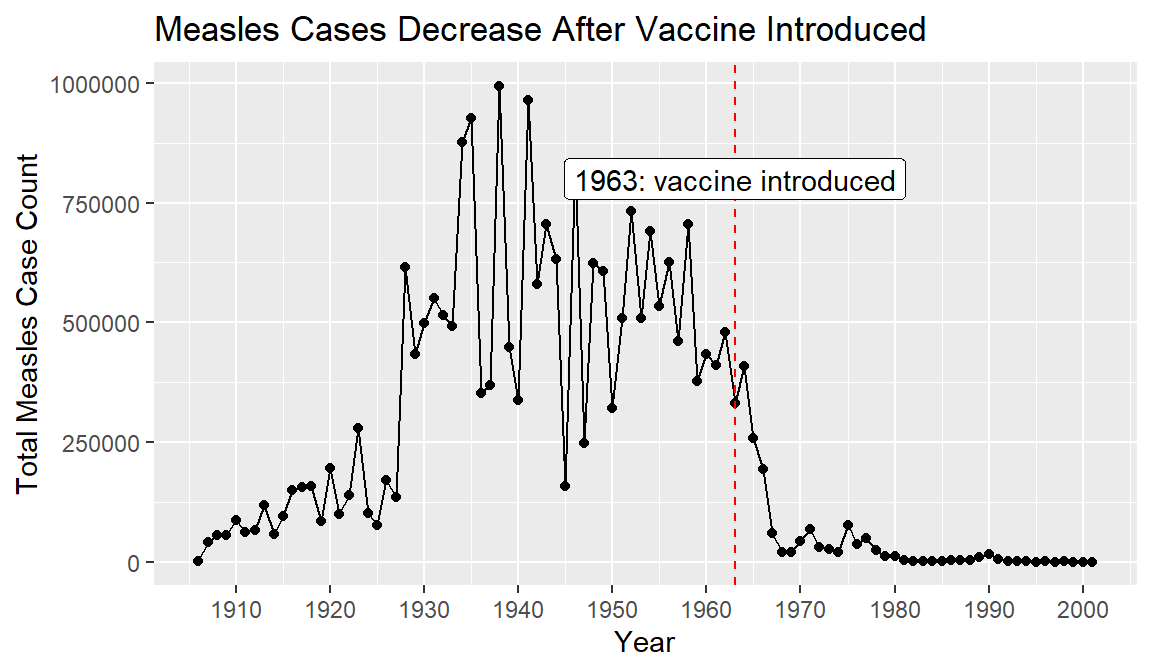Finally, let’s save our plot to a png file, so we can share it or put it in reports. To do this we use the function called `ggsave()`.

``ggsave("images/yearly_measles_count.png", plot = year_total_line)``
``## Saving 6 x 3.5 in image``